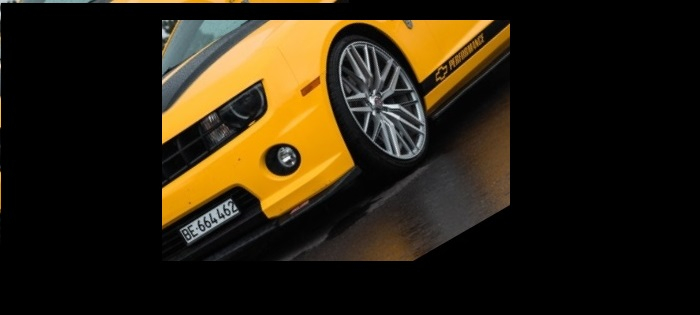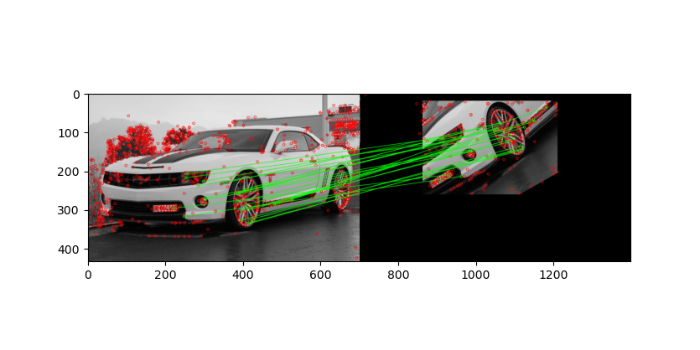# How to implement FLANN based feature matching in OpenCV Python?

We implement feature matching between two images using Scale Invariant Feature Transform (SIFT) and FLANN (Fast Library for Approximate Nearest Neighbors). The SIFT is used to find the feature keypoints and descriptors. A FLANN based matcher with knn is used to match the descriptors in both images. We use cv2.FlannBasedMatcher() as the FLANN based matcher.

## Steps

To implement feature matching between two images using the SIFT feature detector and FLANN based matcher, you could follow the steps given below:

• Import required libraries OpenCV, Matplotlib and NumPy. Make sure you have already installed them.

• Read two input images using cv2.imread() method as grayscale images. Specify the full path of the image.

• Initiate SIFT object with default values using sift=cv2.SIFT_create().

• Detect and compute the keypoints 'kp1' & 'kp2' and descriptors 'des1' & 'des2' in the both input images using sift.detectAndCompute().

• Create a FLANN based matcher object flann = cv2.FlannBasedMatcher() and match the descriptors using flann.knnMatch(des1,des2,k=2). It returns the matches. Apply the ratio test on the matches to obtain best matches. Draw the matches using cv2.drawMatchesKnn().

• Visualize the keypoint matches.

Let's look at some examples to match keypoints of two images using the SIFT feature detector and FLANN based matcher.

## Input Images

We use the following images as input files in the example below.## Example

In this example, we detect the keypoints and descriptors of the two input images using SIFT algorithm and match the descriptors using the FLANN based matcher with knn. Also we apply a ratio test to find the good matches only. We also draw keypoints and matches.

# import required libraries
import numpy as np
import cv2
from matplotlib import pyplot as plt

# Initiate SIFT detector
sift = cv2.SIFT_create()

# find the keypoints and descriptors with SIFT
kp1, des1 = sift.detectAndCompute(img1,None)
kp2, des2 = sift.detectAndCompute(img2,None)

# FLANN parameters
FLANN_INDEX_KDTREE = 0
index_params = dict(algorithm = FLANN_INDEX_KDTREE, trees = 5)
search_params = dict(checks=50) # or pass empty dictionary

# apply FLANN based matcher with knn
flann = cv2.FlannBasedMatcher(index_params,search_params)
matches = flann.knnMatch(des1,des2,k=2)

# Need to draw only good matches, so create a mask
matchesMask = [[0,0] for i in range(len(matches))]

# ratio test as per Lowe's paper
for i,(m,n) in enumerate(matches):
if m.distance < 0.1*n.distance: